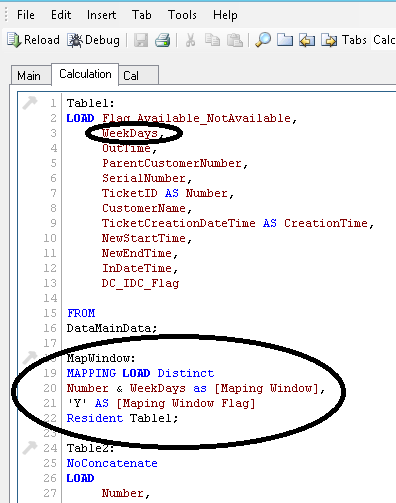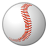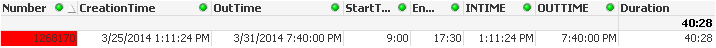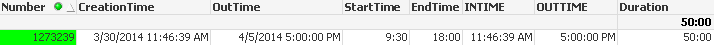# QlikView App Dev

Discussion Board for collaboration related to QlikView App Development.

cancel
Showing results for
Did you mean:Specialist III

## Calculation Error

Hi All

I am doing Calculation of Numbers based on Logic.

The Correct Calculation are Green in Color and Wrong Calculation is Red in Color.

I am calculation Time Difference between CreationTime & OutTime based on:

1. The Creation Time & End Time should be considered between StartTime & EndTime.

2. Eg. 1268170, 1274294, 1300082, 1435122 - Consider only Mon-Fri and exclude Saturday & Sunday.

I have implemented logic of Weekdays in the Logic.Kindly, see the Attachment.

Regards

Av7eN

1 Solution

Accepted SolutionsSpecialist

Again... Cerate 3 columns. I correct the values for you.

Start:

Sum({<[Process Flag]={'START'}>}

If(Timestamp(CreationTime)>Timestamp(Floor(CreationTime)+Time(EndTime)),0,Timestamp(Floor(CreationTime)+Time(EndTime))-Timestamp(CreationTime)))*24*60

Other:

Sum({<[Process Flag]={'OTHER'}>}[Total Number of Working Hours])

End:

Sum({<[Process Flag]={'END'}>}

If(Timestamp(OutTime)>Timestamp(Floor(OutTime)+EndTime),Timestamp(Floor(OutTime)+EndTime),Timestamp(OutTime))-Timestamp(Floor(OutTime)+StartTime))*60*24

Duration:

Interval((([Start] + [Other] + [End]))/24/60, 'hh:mm')

10 RepliesNot applicable

Hi,

Left join(Table1)

Table2:

weekdays,

Weekday(weekdays) as Weeknumb

resident Table1 where Match(weekdays,'Mon','Tue','Wed','Thu','Fri');Specialist

Sometimes when you are trying to solve something and understand the problem is difficult to explain.

Please, give me the exact procedure of how get 38:18 in your qvw and the other red codes.Specialist III
Author

Hi

I am explaining 2 scenarios how the Calculation is done:

Case No.1:It's Creation Time is  25/3/2014 1:11:24 PM

&   OutTime             is   31/3/2014 7:40:00 PM

If you Reload Attached qvd DataMainData, than you'll see its Working weekdays are different for individual Numbers.

For some of the cases Working Weekdays are Mon - Fri

& For some of the cases Working Weekdays are Mon - Sat

It's Working Weekdays are Mon - Fri

Also, calculate difference b/w OutTime & CreationTime by considering Start Time & End Time i.e. different individual Numbers

25 = 4:18:36 hrs ( Hours b/w 9:00 to 17:30)   Calculation=(17:30 - 1:11:24 = 4:18:36)

26 = 8:30 hrs  ( Hours b/w 9:00 to 17:30)   Calculation=(17:30 - 9:00= 8:30:00)

27 = 8:30 hrs  ( Hours b/w 9:00 to 17:30)   Calculation=(17:30 - 9:00= 8:30:00)

28 = 8:30 hrs  ( Hours b/w 9:00 to 17:30)  Calculation=(17:30 - 9:00= 8:30:00)

29 = 0 hrs   ( Saturdayto be excluded since Working Weekdays are Mon - Fri)

30 = 0 hrs  ( Sunday to be excluded since Working Weekdays are Mon - Fri)

31 = 8:30 hrs  ( Hours b/w 9:00 to 17:30)  Calculation=(17:30 - 9:00= 8:30:00)

Sum of All = 4:18:36 hrs + 8:30 hrs + 8:30 hrs + 8:30 hrs + 8:30 hrs = 38:18:36 hrs

Case No.2:It's Creation Time is  30/3/2014 11:46:39 AM

&   OutTime             is   5/4/2014 5:00:00 PM

If you Reload Attached qvd DataMainData, than you'll see its Working weekdays are different for individual Numbers.

For some of the cases Working Weekdays are Mon - Fri

& For some of the cases Working Weekdays are Mon - Sat

It's Working Weekdays are Mon - Sat

Also, calculate difference b/w OutTime & CreationTime by considering Start Time & End Time i.e. different individual Numbers

30 = 0 hrs  ( Sunday to be excluded since Working Weekdays are Mon - Sat)

31 = 8:30 hrs ( Hours b/w 9:30 to 18:00)   Calculation=(18:00 - 9:30 = 8:30:00)

1  = 8:30 hrs ( Hours b/w 9:30 to 18:00)  Calculation=(18:00 - 9:30 = 8:30:00)

2  = 8:30 hrs ( Hours b/w 9:30 to 18:00)   Calculation=(18:00 - 9:30 = 8:30:00)

3  = 8:30 hrs ( Hours b/w 9:30 to 18:00)   Calculation=(18:00 - 9:30 = 8:30:00)

4 = 8:30 hrs ( Hours b/w 9:30 to 18:00)   Calculation=(18:00 - 9:30 = 8:30:00)

5  = 7:30 hrs ( Hours b/w 9:30 to 18:00)   Calculation=(5:00 - 9:30 = 7:30:00)

Sum of All = 8:30 hrs+ 8:30 hrs + 8:30 hrs + 8:30 hrs + 8:30 hrs + 7:30 hrs = 50:00:00 hrsSpecialist III
Author

Any Suggestions?????Specialist

I suggest that you calculate as follows in the script:

1. Days between multiply by the difference of outitme - intime (use the function networkdays)

2. Firstdate - dayend

3. Lastdate - dayend

Sum all, as you describe in your example.

Later i can sent you an example with small data, but now i am very busy.Not applicable

Please do like this for exact calculation.

1. Calculate seconds  b/n 9.30 AM to 6PM  --> 30600

2. Calculate Network days b/n Start Time & End Time & Multiply with working seconds

NetWorkDays( Floor(StartTime) , Floor(EndTime) ) * 30600

3. Then calculate the First day & last day seconds.Specialist

I see your data again, check total number of working hours with the flag "END".  In this case you need to calculate this end hours asking if the OutTime > OUTTIME, because this time for the case is out of the end time.

correct that and check againSpecialist

Again... Cerate 3 columns. I correct the values for you.

Start:

Sum({<[Process Flag]={'START'}>}

If(Timestamp(CreationTime)>Timestamp(Floor(CreationTime)+Time(EndTime)),0,Timestamp(Floor(CreationTime)+Time(EndTime))-Timestamp(CreationTime)))*24*60

Other:

Sum({<[Process Flag]={'OTHER'}>}[Total Number of Working Hours])

End:

Sum({<[Process Flag]={'END'}>}

If(Timestamp(OutTime)>Timestamp(Floor(OutTime)+EndTime),Timestamp(Floor(OutTime)+EndTime),Timestamp(OutTime))-Timestamp(Floor(OutTime)+StartTime))*60*24

Duration:

Interval((([Start] + [Other] + [End]))/24/60, 'hh:mm')Specialist III
Author

Thanks Juan

Your Logic and suggestion worked like a charm.

Thank You very much no.of times. You Made my Day.........................  🙂

Regards

Av7eN